## Friday, 10 October 2014

### CBSE Class 9 - Maths - Quadrilaterals (NCERT Ex 8.2)

NCERT Ex 8.2

Q1: ABCD is a quadrilateral in which P, Q, R and S are mid-points of the sides AB, BC, CD and DA (see the given figure). AC is a diagonal. Show that: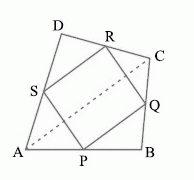(i) SR || AC and SR =AC

(ii)PQ = SR

(iii)PQRS is a parallelogram.

(i) In ▲ADC, S and R are the mid-points of sides AD and CD respectively.

In a triangle, the line segment joining the mid-points of any two sides of the triangle is parallel to the third side and is half of it.

∴ SR || AC and SR =AC ... (1)

(ii) In ▲ABC, P and Q are mid-points of sides AB and BC respectively. Therefore, by using mid-point theorem,

PQ || AC and PQ = AC ... (2)

Using equations (1) and (2), we obtain

PQ || SR and PQ = SR ... (3)

⇒ PQ = SR

(iii) From equation (3), we obtained

PQ || SR and PQ = SR

Clearly, one pair of opposite sides of quadrilateral PQRS is parallel and equal.

Hence, PQRS is a parallelogram.

Q2: ABCD is a rhombus and P, Q, R and S are the mid-points of the sides AB, BC, CD and DA respectively. Show that the quadrilateral PQRS is a rectangle.

In ▲ABC, P and Q are the mid-points of sides AB and BC respectively.

∴ PQ || AC and PQ =AC (Using mid-point theorem) ... (1)

R and S are the mid-points of CD and AD respectively.

∴ RS || AC and RS =AC (Using mid-point theorem) ... (2)

From equations (1) and (2), we obtain

PQ || RS and PQ = RS

Since in quadrilateral PQRS, one pair of opposite sides is equal and parallel to each other, it is a parallelogram.

Let the diagonals of rhombus ABCD intersect each other at point O.

MQ || ON (∵ PQ || AC)

QN || OM (∵ QR || BD)

Therefore, OMQN is a parallelogram.

⇒ ∠MQN = ∠NOM

⇒ ∠PQR = ∠NOM

However, ∠NOM = 90° (Since diagonals of a rhombus are perpendicular to each other)

∴ ∠PQR = 90°

Clearly, PQRS is a parallelogram having one of its interior angles as 90°.

Hence, PQRS is a rectangle.

Q3: ABCD is a rectangle and P, Q, R and S are mid-points of the sides AB, BC, CD and DA respectively. Show that the quadrilateral PQRS is a rhombus.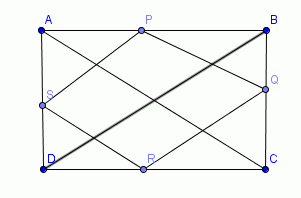Let us join AC and BD.

In ▲ABC,

P and Q are the mid-points of AB and BC respectively.

∴ PQ || AC and PQ =AC (Mid-point theorem) ... (1)

SR || AC and SR =AC (Mid-point theorem) ... (2)

Clearly, PQ || SR and PQ = SR

Since in quadrilateral PQRS, one pair of opposite sides is equal and parallel to each other, it is a parallelogram.

∴ PS || QR and PS = QR (Opposite sides of parallelogram)... (3)

In ▲BCD, Q and R are the mid-points of side BC and CD respectively.

∴ QR || BD and QR =BD (Mid-point theorem) ... (4)

However, the diagonals of a rectangle are equal.

∴ AC = BD ...(5)

By using equation (1), (2), (3), (4), and (5), we obtain

PQ = QR = SR = PS

Therefore, PQRS is a rhombus.

Q4: ABCD is a trapezium in which AB || DC, BD is a diagonal and E is the mid - point of AD. A line is drawn through E parallel to AB intersecting BC at F (see the given figure). Show that F is the mid-point of BC.Let EF intersect DB at G.

By converse of mid-point theorem, we know that a line drawn through the mid-point of any side of a triangle and parallel to another side, bisects the third side.

In ▲ABD,

EF || AB and E is the mid-point of AD.

Therefore, G will be the mid-point of DB.

As EF || AB and AB || CD,

∴ EF || CD (Two lines parallel to the same line are parallel to each other)

In ▲BCD, GF || CD and G is the mid-point of line BD. Therefore, by using converse of mid-point theorem, F is the mid-point of BC.

Q5: In a parallelogram ABCD, E and F are the mid-points of sides AB and CD respectively (see the given figure). Show that the line segments AF and EC trisect the diagonal BD.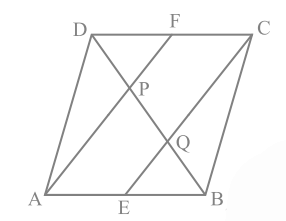ABCD is a parallelogram.

∴AB || CD

And hence, AE || FC

Again, AB = CD (Opposite sides of parallelogram ABCD)AB =CD

AE = FC (E and F are mid-points of side AB and CD)

In quadrilateral AECF, one pair of opposite sides (AE and CF) is parallel and equal to each other.

Therefore, AECF is a parallelogram.

⇒ AF || EC (Opposite sides of a parallelogram)

In ▲DQC, F is the mid-point of side DC and FP || CQ (as AF || EC). Therefore, by using the converse of mid-point theorem, it can be said that P is the mid-point of DQ.

⇒ DP = PQ ... (1)

Similarly, in ▲APB, E is the mid-point of side AB and EQ || AP (as AF || EC). Therefore, by using the converse of mid-point theorem, it can be said that

Q is the mid-point of PB.

⇒ PQ = QB ... (2)

From equations (1) and (2),

DP = PQ = BQ

Hence, the line segments AF and EC trisect the diagonal BD.

Q6: Show that the line segments joining the mid-points of the opposite sides of a quadrilateral bisect each other.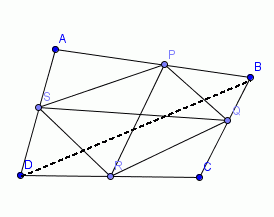Let ABCD is a quadrilateral in which P, Q, R, and S are the mid-points of sides AB, BC, CD, and DA respectively. Join PQ, QR, RS, SP, and BD.

In ▲ABD, S and P are the mid-points of AD and AB respectively. Therefore, by using mid-point theorem, it can be said that

SP || BD and SP =BD ... (1)

Similarly in ▲BCD,

QR || BD and QR =BD ... (2)

From equations (1) and (2), we obtain

SP || QR and SP = QR

In quadrilateral SPQR, one pair of opposite sides is equal and parallel to each other. Therefore, SPQR is a parallelogram.

We know that diagonals of a parallelogram bisect each other.

Hence, PR and QS bisect each other.

Q7: ABC is a triangle right angled at C. A line through the mid-point M of hypotenuse AB and parallel to BC intersects AC at D. Show that

(i) D is the mid-point of AC

(ii) MD ⊥ AC

(iii) CM = MA =AB(i) In ▲ABC,

It is given that M is the mid-point of AB and MD || BC.

Therefore, D is the mid-point of AC.(Converse of mid-point theorem)

(ii)As DM || CB and AC is a transversal line for them, therefore,

∠MDC + ∠DCB = 180º (Co-interior angles)

∠MDC + 90º = 180º

∠MDC = 90º

∴ MD ⊥ AC

(iii) Join MCIn ▲AMD and ▲CMD,

AD = CD (D is the mid-point of side AC)

∠ADM = ∠CDM (Each 90º)

DM = DM (Common)

∴▲AMD ≅ ▲CMD (By SAS congruence rule)

Therefore, AM = CM(By CPCT)

However, AM =AB (M is the mid-point of AB)

Therefore, it can be said that

CM = AM =AB1.Heylo how do you do ... I am fine thank to you

2.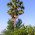very nice. give some more examples

3.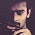useful ;) !!
keep postin' :)

4.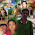need some more

5.need some more

6.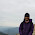Egg biryani!! very helpful

7.i need more .here it is given what ever is there already in the text

8.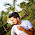it was nice
9.